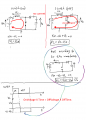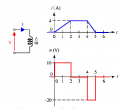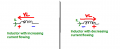# Boost converter - Inductor Polarity

#### eedrive

Joined Jan 29, 2021
6
Hello,

I have a question about boost converter.
I don't understand why in the off-state the waveforms (also at wikipedia) showing that
the voltage of the inductor is VL = Vin - Vo. I understand that in the off-state the inductor act like a source
and his polarity is changed. if so, the KVL should be different and gives that VL = Vo - Vin
and not VL = Vin - Vo. If I'm analyzing the waveforms it's looks like the KVL means that the inductor still consider as a consumer and not as a source.
But I read that the inductor change his polarity in the off-state.. So how it's make sense .. Where I'm wrong ?

Hope you can help,

#### ronsimpson

Joined Oct 7, 2019
2,609
I draw current as + to - flow, many people say - to +. Really does not matter.
When the switch is closed there is no current going to the output capacitor. Current is in L.
When the switch is open the current pushes up into the diode and Cout.

The On Time X Vin = Off Time X Vout-Vin.
When switch is closed there is (voltage X time) on the inductor.
When the switch is open there is (voltage X time) on the inductor in the opposite direction.
The voltage x time is no measured from ground but across the inductor.#### crutschow

Joined Mar 14, 2008
31,511
The mechanical analog of an inductance is inertia.

So the inductor current will always try to stay flowing in the same direction, and it will generate whatever voltage and polarity is needed to maintain that current (until all the inductive energy is dissipated).
Thus, when you apply a voltage from the left (positive) to the right (negative) across an inductor, its current will increase in a left-to-right direction as determined by the voltage and inductance.
When the voltage is removed, the inductance will try to keep the current moving in the same direction, making the inductor now act as a voltage source, with the right terminal positive and the left terminal negative.

In summary, the voltage across the inductor changes between charging and discharging, but the current keeps moving in the same direction.

Last edited:

#### BobTPH

Joined Jun 5, 2013
6,298
This is all encoded in the inductor equation:

V = L dI / dt

You can see that when the current is increasing, the voltage is positive and when it is decreasing, it is negative.

Bob

•eedrive

#### eedrive

Joined Jan 29, 2021
6
I draw current as + to - flow, many people say - to +. Really does not matter.
When the switch is closed there is no current going to the output capacitor. Current is in L.
When the switch is open the current pushes up into the diode and Cout.

The On Time X Vin = Off Time X Vout-Vin.
When switch is closed there is (voltage X time) on the inductor.
When the switch is open there is (voltage X time) on the inductor in the opposite direction.
The voltage x time is no measured from ground but across the inductor.
View attachment 229005
Hey, thanks for your reply. Sorry for the mistake showing an arrow of current in the left drawing. It's clear to me that there is no current in the places you marked. But I still don't understand why VL is equal to Vi - Vo and not Vi - Vo. This was my question. The balance equation is clear to me. I understand the theory. In terms of math, it does not work out for me. You said that: "The voltage x time is no measured from ground but across the inductor. " why is that ? no current is clear .. but there is still voltage, so what is the correct kvl ? I don't get it... thanks again.

#### eedrive

Joined Jan 29, 2021
6
The mechanical analog of an inductance is inertia.

So the inductor current will always try to stay flowing in the same direction, and it will generate whatever voltage and polarity is needed to maintain that current (until all the inductive energy is dissipated).
Thus, when you apply a voltage from the left (positive) to the right (negative) across an inductor, its current will increase in a left-to-right direction as determined by the voltage and inductance.
When the voltage is removed, the inductance will try to keep the current moving in the same direction, making the inductor now act as a voltage source, with the right terminal positive and the left terminal negative.

In summary, the voltage across the inductor changes between charging and discharging, but the current keeps moving in the same direction.
Thanks for all the theoretical explanation, but still something not work out for me from the math aspect as I mentioned :\

#### eedrive

Joined Jan 29, 2021
6
This is all encoded in the inductor equation:

V = L dI / dt

You can see that when the current is increasing, the voltage is positive and when it is decreasing, it is negative.

Bob
This is all encoded in the inductor equation:

V = L dI / dt

You can see that when the current is increasing, the voltage is positive and when it is decreasing, it is negative.

Bob
Yes true, but why the kvl is not vin - vo + vl = 0 --> vl = vo - vin ?
it's should be like that if i consider that the inductor is changing polarity. same as my attachment.
but in the waveform it's vl = vin - vo

#### eedrive

Joined Jan 29, 2021
6
Hey, thanks for your reply. Sorry for the mistake showing an arrow of current in the left drawing. It's clear to me that there is no current in the places you marked. But I still don't understand why VL is equal to Vi - Vo and not Vi - Vo. This was my question. The balance equation is clear to me. I understand the theory. In terms of math, it does not work out for me. You said that: "The voltage x time is no measured from ground but across the inductor. " why is that ? no current is clear .. but there is still voltage, so what is the correct kvl ? I don't get it... thanks again.
I mean, in off state Vo = Vin + VL ( two sources in series ) same as VL = Vo - Vin
So why in the graph it's mentioned Vin - Vo

#### BobaMosfet

Joined Jul 1, 2009
2,063
Hey, thanks for your reply. Sorry for the mistake showing an arrow of current in the left drawing. It's clear to me that there is no current in the places you marked. But I still don't understand why VL is equal to Vi - Vo and not Vi - Vo. This was my question. The balance equation is clear to me. I understand the theory. In terms of math, it does not work out for me. You said that: "The voltage x time is no measured from ground but across the inductor. " why is that ? no current is clear .. but there is still voltage, so what is the correct kvl ? I don't get it... thanks again.
Whenever you measure voltage, you are NOT measuring what voltage exists, but what voltage was consumed/lost/dropped across whatever it is you are measuring. Voltage is not present, only where current is either A) not flowing, or B), the current flow is the same at both points being measured. In a buck converter, when the switch is closed, the inductor has virtually no impedance to prevent it from unloading as fast as it can, which causes a current dump, and a voltage spike (up to several times that of the source voltage). In this way, with the help of the capacitor, voltage and current can be 'boosted' for use as a very careful balance between load and source.

•eedrive

#### Jony130

Joined Feb 17, 2009
5,439

In short:
As you should know the voltage across the coil is proportional to the rate of change in current
V = L * dI/dtThis equation indicates that inductance voltage depends not on the current which actually flows through the inductance, but on its rate of change. This means that to produce the voltage across an inductance, the applied current must change. If the current is kept constant, no voltage will be induced, no matter how large the current. Conversely, if it is found that the voltage across an inductance is zero this means that the current must be constant but not necessary zero.

In summary:

When the inductor current increasing (charging phase)the rate of change is positive di/dt > 0, thus, Voltage across the inductor must be positive because L times a positive number yields a positive voltage.

But when the current inductor current starts to decreasing (discharging phase), the rate of change is negative di/dt < 0, so the voltage across the inductor must be negative because L times a negative number yields a negative voltage.#### eedrive

Joined Jan 29, 2021
6

In short:
As you should know the voltage across the coil is proportional to the rate of change in current
V = L * dI/dt
View attachment 229018

This equation indicates that inductance voltage depends not on the current which actually flows through the inductance, but on its rate of change. This means that to produce the voltage across an inductance, the applied current must change. If the current is kept constant, no voltage will be induced, no matter how large the current. Conversely, if it is found that the voltage across an inductance is zero this means that the current must be constant but not necessary zero.

In summary:

When the inductor current increasing (charging phase)the rate of change is positive di/dt > 0, thus, Voltage across the inductor must be positive because L times a positive number yields a positive voltage.

But when the current inductor current starts to decreasing (discharging phase), the rate of change is negative di/dt < 0, so the voltage across the inductor must be negative because L times a negative number yields a negative voltage.

View attachment 229021
Thanks for that ! The waveforms really helps. In the part where di/dt should be negative that's makes things more clear.
When thinking only from the math aspect like doing kvl, it's really easy to confuse what really happen.

#### matthow

Joined Nov 10, 2020
2
Yes true, but why the kvl is not vin - vo + vl = 0 --> vl = vo - vin ?
it's should be like that if i consider that the inductor is changing polarity. same as my attachment.
but in the waveform it's vl = vin - vo
Hi eedrive.
You might want to have a look at this page from imperix, which explains the theory and operation of buck and boost converter structures: https://kb.imperix.com/pages/viewpage.action?pageId=787663 There are simulation models attached.
Hope it helps.